# Decimals Class 6 Worksheet

Please check Solutions of Decimals Class 6 Worksheet at the end of the questions.1. Find whole-number part and decimal part from 78.9
a) Whole number part = 78 , Decimal part = 9
b) Whole number part = 9 , Decimal part = 78

2. Find the unlike decimal in the given sets of decimals?
a) 3.28
b) 2.5
c) 3.51
d) 1.61

3. Fill the missing place: 20.97 ______ 17.33
a) <
b) >

4. Fill the missing place: 2.87 _______ 2.34
a) <
b) >

5. Convert 0.2 into a fractions in its Simplest form?
a) 1/6
b) 3/5
c) 1/5
d) 1/7

6. Write the place value of 4 in 3476.568
a) 4/10
b) 4/100
c) 40
d) 400

7. Express 5 kg 265 g in kg.
a) 526.5 kg
b) 5.265 kg
c) 52.65 kg
d) 5.0265 kg

8. Express 7 km 475 m in km?
a) 11.75 km
b) 7.0475 km
c) 54.5 km
d) 7.475 km

9. Express ₹ 36 and 77 paise into rupees.
a) ₹ 36.0077
b) ₹ 36.77
c) ₹ 367.7
d) ₹ 36.077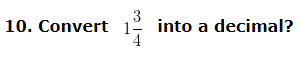a) 1.3
b) 1.075
c) 1.5
d) 1.75

11. Add 87.4, 78, 352.9 and 234.282
a) 744.612
b) 752.582
c) 769.362

12. Subtract 71.41 from 83.2
a) 10.79
b) 11.79
c) 12.59

13. What should be added to 64.73 to get 70
a) 3.27
b) 5.27
c) 5.37
d) 4.37

14. What is to be subtracted from 8.7 to get 5.3
a) 2.1
b) 3.4
c) 4.7
d) 4.4

15. Arrange the following decimals in ascending order 5.24 , 5.73 and 8.26
a) 8.26 < 5.73 < 5.24
b) 5.24 < 5.73 < 8.26
c) 5.24 < 8.26 < 5.73

### Decimals Class 6 Worksheet Solutions

Decimals Class 6 Worksheet – Solution 1

Decimal has two parts; whole number part and decimal part and these parts are separated by a dot (.)
The left side of decimal point is called whole number part
Hence, the whole number part in the given number is = 78
The right side of decimal point is called decimal part
Hence, the decimal part in the given number is = 9

Correct Answer – a) Whole number part = 78 , Decimal part = 9

Decimals Class 6 Worksheet – Solution 2

Decimal having the same number of decimal places are called Like Decimals
The number of decimal places in 3.28 is 2
The number of decimal places in 2.5 is 1
The number of decimal places in 3.51 is 2
The number of decimal places in 1.61 is 2
Hence, 2.5 is unlike decimal since it has only 1 decimal place while all other numbers have 2 decimal places

Decimals Class 6 Worksheet – Solution 3

The given decimal are 20.97 and 17.33
When we compare the decimals, first step is to compare the whole-number part of the two decimals. If the whole number part is the same, then we compare the decimal part. Else the decimal with the higher whole number part is the larger decimal
Let us compare their whole – number parts
The left side of decimal point is called whole number part
The Whole number part of the two numbers are 20 and 17
Clearly, 20 > 17
Hence, 20.97 > 17.33

Decimals Class 6 Worksheet – Solution 4

Let us compare their whole number parts
The left side of decimal point is called whole number part
The whole number part of 2.87 and 2.34 are equal that is 2
Let us compare their tenth digits
The first digit after decimal point is called tenth place value
The tenth place value of the two numbers are 0.8 0.3
Clearly, 0.8 > 0.3
Since, 2.87 > 2.34

Decimals Class 6 Worksheet – Solution 5

Step 1: Write the given decimal without the decimal point as the numerator of the fraction = 2
Step 2: Denominator, write 1 followed by as many zeroes, as there are decimal places in the given decimal = 10
= 2/10
Step 3: Convert the fraction 2/10 into simplest form
HCF of 2 and 10 is 2
then, 2/10 = (2÷2)/(10÷2) = 1/5 (To simplify this we have to divide numerator and denominator by HCF of 2 and 10 i.e 2 )
Hence, 0.2 = 1/5

Decimals Class 6 Worksheet – Solution 6

Place value of 4 = 4 Hundreds = 400

Decimals Class 6 Worksheet – Solution 7

First, we have to convert 265 g into kg
As we know that, 1000 g = 1 kg
1 g = 1/1000 kg
265 g = 1/1000 x 265 kg
265 g = 265/1000 kg
265 g = 0.265 kg
Now we have to convert, 5 kg 265 g into kg
5 kg + 265 g
= 5 kg + 0.265 kg (From above 265 g = 0.265 kg )
= 5.265 kg

Correct Answer – b) 5.265 kg

Decimals Class 6 Worksheet – Solution 8

First, we have to convert 475 m into km
As we know that, 1000 m = 1 km
1 m = 1/1000 km
475 m = ( 1/1000 x 475 ) km
475 m = 475/1000 km
475 m = 0.475 km
Now we have to convert, 7 km 475 m into km
7 km + 475 m
= 7 km + 0.475 km (From above 475 m = 0.475 km )
= 7.475 km

Correct Answer – d) 7.475 km

Decimals Class 6 Worksheet – Solution 9

As we know that, 100 paise = 1 rupees
1 paise = 1/100 rupees
77 paise = ( 1/100 x 77 ) rupees
77 paise = ₹ 77/100
77 paise = ₹ 0.77
Now we have to convert, ₹ 36 and 77 paise into rupees
₹ 36 + 77 paise
= ₹ 36 + ₹ 0.77 (From above 77 paise = ₹ 0.77 )
= ₹ 36.77

Correct Answer – b) ₹ 36.77

Decimals Class 6 Worksheet – Solution 10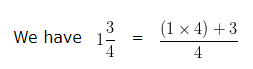= (4+3)/4
= 7/4
On dividing 7 by 4 we get 1.75
Hence, the decimal value of the given fraction is 1.75

Decimals Class 6 Worksheet – Solution 11

On Converting the given decimals into like decimals, we get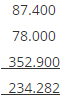Writing these decimals in column form and adding ,we get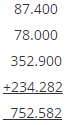Hence, the sum of the given decimal is 752.582

Decimals Class 6 Worksheet – Solution 12

On Converting the given decimals into like decimals, we get 71.41 and 83.20

Writing these like decimals in column form with the largest one at the top and then subtracting , we get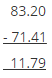Hence, On subtracting 71.41 from 83.2 we get 11.79

Decimals Class 6 Worksheet – Solution 13

Lets say the number to be added to 64.73 to get 70 is x
This implies that 64.73 + x = 70
or x = 70 – 64.73
Thus, in this question we have to subtract 64.73 from 70 to get the number which should be added to 64.73 to become 70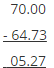Hence, 5.27 is to be added to 64.73 to become 70

Decimals Class 6 Worksheet – Solution 14

Lets say the number to be subtracted to 8.7 to get 5.3 is x
This implies that 8.7 – x = 5.3
or x = 8.7 – 5.3
Thus, in this question we have to subtract 5.3 from 8.7 to get the number which should be subtracted to 8.7 to become 5.3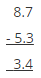Hence, 3.4 is to be subtracted to 8.7 to become 5.3

Decimals Class 6 Worksheet – Solution 15

Ascending order means to arrange the number from smaller number to greater number
Place Value Chart: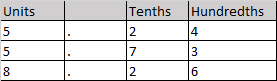When we compare the decimal, first compare the whole-number part and then compare the decimal part.
When we compare the whole-number part we find that 8 is greater than 5 and 5
Hence 5.24 and 5.73 < 8.26
Between 5.24 and 5.73 since whole number part is same, we compare the decimal parts
Clearly 5.24 < 5.73
Hence, decimal numbers in ascending​ order are: 5.24 < 5.73 < 8.26

Correct Answer – b) 5.24 < 5.73 < 8.26